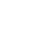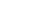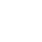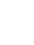# How Does Simple Interest Differ From Compound Interest?

## Understanding the differences between simple and compound interest can help you save when you borrow money.Contributing Writer at Tally

June 29, 2021

A recent study by CreditCards.com found that 48% of Americans are either unsure or unaware of their credit card annual percentage rates (APRs). If you have — or are thinking of opening — a credit card, it's important to understand how interest rates work.

To better understand credit card interest rates, you should start by comparing simple vs. compound interest. Today, we're here to answer the question, "How does simple interest differ from compound interest?" By the end of this article, you should have a much better understanding of the differences between the two and how they impact you when borrowing money.

## What is an Interest Rate?

In the simplest of terms, an interest rate is an amount a lender charges you to borrow money. An interest rate is expressed as a percentage of the principal borrowed. Interest rates typically apply when you borrow money for things like:

As we will explain below, there are two different types of interest: simple and compound.

## What is Simple Interest?

Simple interest is an interest charge that only applies to the principal amount of the loan. You can quickly determine the total amount of interest owed by using the following formula:

Amount of simple interest owed = P × r × n

In this equation:

• P = principal amount of a loan

• r = annual interest rate

• n = duration of the loan expressed in

number of years

Let's say that you borrow \$20,000 from a financial institution. You and the lender agree to a simple interest rate of 5% with a loan term of 10 years. You can calculate the amount you will owe by using the simple interest rate formula:

\$20,000 × .05 × 10 = \$10,000

This means that you will owe \$10,000 in total interest. If you add this to your initial principal of \$20,000, the total amount of money you owe is \$30,000.

Calculating this figure before you agree to terms with a lender is useful because it allows you to project your future cash flow better. While you may think you’re getting a good deal with a 5% interest rate, you quickly realize that you will end up paying not only the principal but also an additional 50% of the principal balance in interest alone.

That being said, simple interest is straightforward because you can easily determine how much you will owe. As we will discover below, calculating compound interest is a bit more complicated.## What is Compound Interest?

Compound interest accumulates interest on itself.  Compounded interest snowballs and is more difficult to project because interest accrues its own interest. Not only do you owe the amount of the loan, but you also owe any interest that accumulates.

Let's start by looking a the compound interest formula:

Compound Interest = P[(1+r)^n – 1]

In this equation:

• P = total principal

• r = interest rate

• n = the number of compounding periods, expressed in years

Consider the following compound interest example, using the same figures as our simple interest loan. If you recall, you borrowed \$20,000 from a financial institution. You and the lender agreed to a 5% rate of interest. The defined period of time on the loan is 10 years.

\$20,000[(1+.05)^10 – 1] = \$20,000(.6289) = \$12,578

The accumulated interest is \$12,578. You owe this on top of your principal amount of \$20,000. The total amount that you owe is \$32,578. The amount is higher than the \$10,000 you owe in the simple interest example because compound interest collects interest on itself.

## How is Compound Interest Different from Simple Interest?The key difference between compound interest and simple interest is how lenders determine what you owe.

With simple interest, you know exactly how much you owe over the life of the loan. The interest is calculated based on the principal only. The amount is fixed, which makes it easier to project from a cash flow perspective. Mortgages and car loans often use simple interest.

Compound interest, on the other hand, builds on itself. The interest is calculated not only on the principal but also on your total balance, including existing interest. You are charged interest on top of interest. A credit card is an example of a lending option that typically has compounding interest.

Compounding interest can grow your total balance quickly. If all other terms are the same, you'll end up paying more in compound interest than you would in simple interest. Simple interest tends to be the most affordable option for borrowers, meaning it's the better option to save money on interest

## What are Some of the Common Misunderstandings Regarding Simple and Compound Interest?

One of the biggest misconceptions about compound interest that people don't realize is that the interest is added to your balance. For instance, let's say that you have a \$100 balance on your credit card. Your lender charges you \$5 in interest. The next month, your interest charge is determined not on \$100 but \$105. Your next interest charge will be higher because the balance is higher.

A common misconception about simple interest is that it cannot change. It's true that the interest charge is fixed in that it only applies to the principal. However, if you agree to a variable APR, your rate can still fluctuate.

For instance, let's say you have a 5% interest rate applied to a \$100 principal every month, so you owe \$105 per month. Your rate can increase or decrease with the Fed funds rate. If your rate increases to, say, 7%, you would owe \$107 per month. However, this interest will always apply to your principal balance only because it is a simple interest rate.

## Understand Simple vs. Compound Interest to Improve Your Personal FinancesAs a borrower, it's important to understand the type of interest your lender applies to your account. Knowing whether you have a simple interest rate or compounding interest rate directly impacts the total amount you owe when borrowing money.

Simple interest is most commonly seen on mortgages and loans. Simple interest is often a better option for the borrower, as they can clearly understand and plan for their monthly payment.

Compounding interest, on the other hand, is a bit more complicated. Often seen in credit cards, lenders charge interest on top of interest if you have a compounding rate. Because of this, you could accumulate debt faster, which is why credit cards can be a risky borrowing option.

If you have collected credit card debt and are looking for a more affordable way to pay it down, consider using Tally. Tally offers a line of credit1 with a lower interest rate that you can use to pay off your credit card debt quickly and efficiently.

`1`To get the benefits of a Tally line of credit, you must qualify for and accept a Tally line of credit. The APR (which is the same as your interest rate) Will be between 7.90% - 29.99% per year, and will be based on your credit history. The APR will vary with the market based on the Prime Rate.